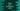# Kotlin program to print the Fibonacci series# Introduction :

In this tutorial, we will learn how to print the Fibonacci series in Kotlin. The Fibonacci series is a series of numbers where each value is the sum of its two preceding values. For example, 0,1,1,2,3,5,8,13 is the Fibonacci series of size 8. We will write programs to print the Fibonacci series of different length i.e. it will take the size and print out the series of that size.

## Using a loop :

You can use any loop to build one Fibonacci series. The below example uses one for loop :

``````fun getFibonacci(size: Int) : MutableList<int>{
val seq = mutableListOf(0)

if(size == 1) return seq

seq.add(1)
for(i in 1 until size-1){
seq.add(seq[seq.lastIndex] + seq[seq.lastIndex - 1])
}

return seq
}

fun main() {
print(getFibonacci(10))
}``````

Here, getFibonacci function takes the series size and returns one mutable Fibonacci series. In this function, we are creating one mutable list seq with value 0. If the value of size is 1, return it else add 1. Now, use one for loop and add the Fibonacci values one by one. i.e. add the sum of last and second last elements to the list. Finally, return this list.

The above program will print :

``[0, 1, 1, 2, 3, 5, 8, 13, 21, 34]``

## Recursive method :

Recursive is a way to call the same method, again and again, to find out a result. We need to get the Fibonacci series. For that, we can create one function that takes the last, second last and one final result list as its parameter. It will keep building the final result list by appending the Fibonacci values. We will call the same method recursively with updated values to create the result.

``````fun getFibonacci(count: Int, first: Int = 0, second: Int = 1, result: MutableList<Int> = mutableListOf()): MutableList<Int> {
return if (count > 0) {
result.add(first)
getFibonacci(count - 1, second, first + second, result)
} else {
result
}
}

fun main() {
print(getFibonacci(5))
}``````

It will print :

``[0, 1, 1, 2, 3]``

Here, getFibonacci function is called recursively. first and second are the second last and last elements of the current Fibonacci series. Initial values are 0 and 1 for these two parameters. count is used to indicate the total numbers to add. On each recursive call, its value is decremented by 1. If its value is greater than 0, we are adding the second last element to result and calling the same method recursively. the result is a mutable list and it is returned once the value of count reaches 0.

## Using sequence and yield :

We can create one Fibonacci list using sequence and yield. Basically, we are creating one Pair with initial two numbers of the Fibonacci series and yield the first element. Update the Pair with the next two values of the Fibonacci series and yield again.

``````fun getFibonacci() = sequence {
var item = Pair(0, 1)

while (true) {
yield(item.first)
item = Pair(item.second, item.first + item.second)
}
}

fun main() {
println(getFibonacci().take(7).toList())
}``````

It will print :

``[0, 1, 1, 2, 3, 5, 8]``

## Using generateSequence :

generateSequence and Pair can also be used to create one Fibonacci series. We need to map the first element each time like below :

``````fun getFibonacci() : Sequence<Int>{
return generateSequence(Pair(0,1),{Pair(it.second, it.first + it.second)}).map{it.first}
}

fun main() {
println(getFibonacci().take(7).toList())
}``````

It will print :

``[0, 1, 1, 2, 3, 5, 8]``